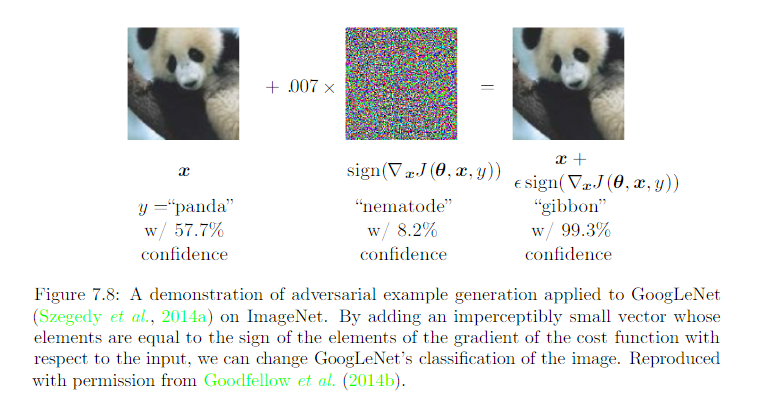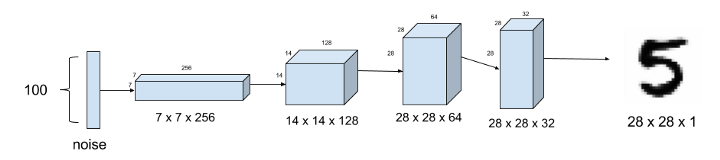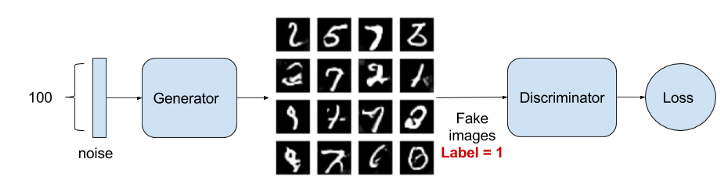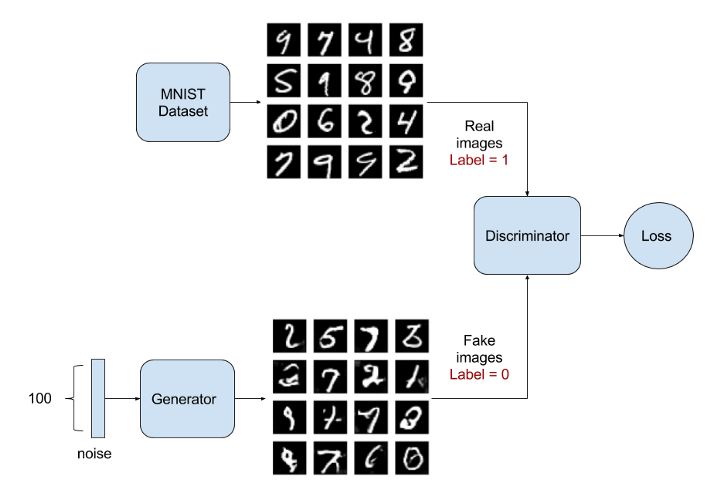# Topics¶

Data inputs that fool neural networks, but not people(image: Deep Learning, Ian Goodfellow and Yoshua Bengio and Aaron Courville)

In this walkthrough, we will see how a Neural Network handles adversarial examples.

1. Get predictions for an image
2. Convert image to an adversarial example

### Setup - Install Foolbox¶

Foolbox is a Python toolbox to create adversarial examples that fool neural networks.

https://github.com/bethgelab/foolbox

pip install foolbox
In [ ]:
def resize_and_crop_image(image_path, width, height):
"""Resizes and crops an image to the desired size
Args:
image_path: path to the image
width: image width
height: image height
Returns:
the resulting image
"""
from PIL import Image, ImageOps

img = Image.open(image_path)
img = ImageOps.fit(img, (width, height))
return img

In [ ]:
# 1. Get predictions for an image
from keras.applications import ResNet50
from keras.applications.resnet50 import preprocess_input, decode_predictions
from keras.preprocessing.image import img_to_array

import matplotlib.pyplot as plt
import numpy as np

model = ResNet50()
width = height = 224

img = resize_and_crop_image(image_path, width, height)
plt.imshow(img)

x = img_to_array(img)
x = preprocess_input(x)
x = np.expand_dims(x, axis=0)
y = model.predict(x)
preds = decode_predictions(y, top=1)
plt.title('Original: %s' % preds)
plt.axis('off')
plt.show()

In [ ]:
from keras.backend import set_learning_phase
from PIL import Image
import foolbox

# labels from Keras
# https://s3.amazonaws.com/deep-learning-models/image-models/imagenet_class_index.json
label = 829 # "829": ["n04335435", "streetcar"]

# Example from: https://github.com/bethgelab/foolbox
set_learning_phase(0) # not training

# Element-wise preprocessing of input
#   first subtracts the first element of preprocessing from the input
#   and then divide the input by the second element.
preprocessing = (np.array([104, 116, 123]), 1)
fmodel = foolbox.models.KerasModel(ResNet50(), bounds=(0, 255),
preprocessing=preprocessing)

# Apply attack on source image to target a different label
attack = foolbox.attacks.FGSM(model=fmodel)

img = resize_and_crop_image(image_path, width, height)
x = np.asarray(img, dtype=np.float32)
x = x[:, :, :3]

# ::-1 to convert BGR to RGB
adversarial = attack(x[:, :, ::-1], label)

# ::-1 to convert BGR to RGB
# division by 255 to convert [0, 255] to [0, 1]

y = model.predict(np.expand_dims(x, axis=0))
preds = decode_predictions(y, top=1)

plt.axis('off')
plt.show()


## Optional Exercises¶

The Foolbox tool kit has a few other exploits available. These are useful if we want to create adversarial inputs to augment our training data (https://github.com/bethgelab/foolbox/issues/81)

1. Try other attacks available in Foolbox, such as LBFGSAttack, which tries to fake a target class.
criterion = foolbox.criteria.TargetClass(22)
attack    = foolbox.attacks.LBFGSAttack(fmodel, criterion)
1. Try other image classes as practice. For a given text label, you can find the integer label by download this file:

• Train two networks against each other
• Generator: generates fake images to fool Discriminator
• returns samples
• Discriminator: tries to distinguish real images from fake ones
• returns probability that sample is real

## Generator¶## Discriminator¶## Objective¶

Training is done (converged) when:

• Generator's fake samples are indistinguishable from real samples
• Discriminator always returns $\frac{1}{2}$

Discard Discriminator and keep the Generator as the finished model.

## Applications¶

Image synthesis: https://arxiv.org/abs/1803.04469

Text to Speech: https://github.com/r9y9/gantts

## Workshop: Training a DCGAN¶

Deep Convolutional GAN: https://arxiv.org/abs/1511.06434

In [ ]:
from keras.layers import Input, Dense, Reshape, Flatten, Dropout
from keras.layers import BatchNormalization
from keras.models import Sequential, Model
import numpy as np
import matplotlib.pyplot as plt


### Dataset: MNIST¶

Training GANs is tricky, so we will try to reproduce it with a well-known dataset (MNIST).

Input: 28x28 pixel, black and white images of handwritten digits

Ouptut: 10 labels (0 to 9)

http://yann.lecun.com/exdb/mnist/

In [ ]:
from keras.datasets import mnist

width = height = 28
channels = 1
shape = (width, height, channels)

(X_train, _), (_, _) = mnist.load_data()

# Rescale -1 to 1
X_train = (X_train.astype(np.float32) - 127.5) / 127.5
X_train = np.expand_dims(X_train, axis=3)


### Create models¶

In [ ]:
def generator():
"""Defines a Generator model"""
model = Sequential()
model.add(Dense(height * height * channels, activation='tanh'))
return model

In [ ]:
def discriminator():
"""Defines a Discriminator model"""
model = Sequential()
model.add(Dense((width * height * channels), input_shape=shape))
return model


The adversarial model is created by chaining the generator with the discriminator.

1. Input goes into the Generator, which tries to make it fake
2. The output from the Generator will be fed into the Discriminator, which tries to discriminate the fake images from real ones.## Exercise - Create Adversarial Model¶

Create our stacked adversarial model as shown in the picture above.

Steps

1. Create and compile the generator with binary_crossentropy loss and Adam(lr=0.0002, decay=8e-9) optimizer

2. Create and compile the discriminator with binary_crossentropy loss and Adam(lr=0.0002, decay=8e-9) optimizer

3. Chain the two into a Sequential() adversarial model, and compile it.

• For the adversarial model, the discriminator's weights should be frozen.

You can refer to https://medium.com/@mattiaspinelli/simple-generative-adversarial-network-gans-with-keras-1fe578e44a87 if you are stuck.

In [ ]:
# Your code here


## Train the GAN¶In [ ]:
def plot_images(samples=16, step=0):
"""Plots the generated images at the given step
Args:
samples: number of images to generate
step: step count
"""
import matplotlib.pyplot as plt

noise = np.random.normal(0, 1, (samples,100))
images = gen.predict(noise)

plt.figure(figsize=(10,10))

for i in range(images.shape):
plt.subplot(4, 4, i+1)
image = images[i, :, :, :]
image = np.reshape(image, [height, width])
plt.imshow(image, cmap='gray')
plt.axis('off')
plt.tight_layout()
plt.show()


### Training Setup: Sanity Check¶

To make sure our training code works, we'll do a sanity check with very few epochs and a tiny batch size.

In [ ]:
epochs = 20 # small number for workshop purposes, typically 20000
batch = 4 # small number for workshop purposes, typically 32
plot_interval = 200

for cnt in range(epochs):

# Get real images
random_index =  np.random.randint(0, len(X_train) - batch//2)
legit_images = X_train[random_index : random_index + batch//2].reshape(batch//2, width, height, channels)

# Have the generator predict fake images
print('epoch: %d, [Generating images, batch size: %d]' % (cnt, batch))
gen_noise = np.random.normal(0, 1, (batch//2,100))
synthetic_images = gen.predict(gen_noise)
x_combined_batch = np.concatenate((legit_images, synthetic_images))
y_combined_batch = np.concatenate((np.ones((batch//2, 1)), np.zeros((batch//2, 1))))

# Train the discriminator with the fake images and the real images
# perform 1 gradient update on this batch
print('epoch: %d, [Training Discriminator, batch size: %d]' % (cnt, batch))
dis_loss = dis.train_on_batch(x_combined_batch, y_combined_batch)

# Train the generator (which is embedded in the Adversarial network)
# For the Adversarial network, the discriminator weights are frozen.
noise = np.random.normal(0, 1, (batch,100))
y_mislabeled = np.ones((batch, 1))

# perform 1 gradient update on this batch
print('epoch: %d, [Training Generator, batch size: %d]' % (cnt, len(x_combined_batch)))
gan_loss = gan.train_on_batch(noise, y_mislabeled)
print('epoch: %d, [Discriminator loss: %.3f], [ Generator loss: %.3f]' % (cnt, dis_loss, gan_loss))

# show progress
if cnt % plot_interval == 0 :
plot_images(step=cnt)


## Exercise - Train model¶

Now that we've run through a quick sanity check, try setting epochs and batch to larger values

epochs = 20 # small number for workshop purposes, typically 20000
batch = 4 # small number for workshop purposes, typically 32

Warning: training will be slow on CPU-only machines. You can try gradually bumping up the epochs / batch values.

Another option is to look into running this on a GPU machine, using a service such as: https://neptune.ml/